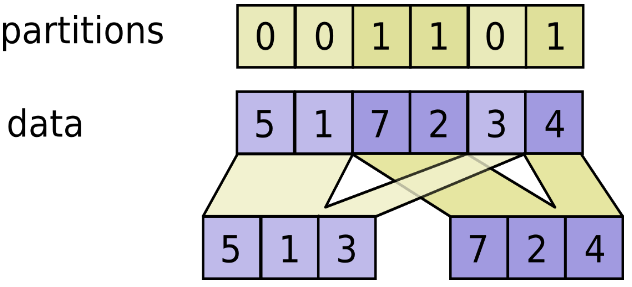# TensorFlow分区索引的使用

## tf.dynamic_partition

``````dynamic_partition(
data,
partitions,
num_partitions,
name=None
)``````

``````outputs[i].shape = [sum(partitions == i)] + data.shape[partitions.ndim:]

outputs[i] = pack([data[js, ...] for js if partitions[js] == i])``````

data.shape 必须和 partitions.shape 一起启动.

``````# Scalar partitions.
partitions = 1
num_partitions = 2
data = [10, 20]
outputs = []  # Empty with shape [0, 2]
outputs = [[10, 20]]

# Vector partitions.
partitions = [0, 0, 1, 1, 0]
num_partitions = 2
data = [10, 20, 30, 40, 50]
outputs = [10, 20, 50]
outputs = [30, 40]``````### ARGS：

• data：一个 Tensor.
• partitions：一个 int32 类型的张量.可以是任何形状.索引在范围 [0, num_partitions) 内.
• num_partitions：一个大于等于1的整数；要输出的分区数.
• name：操作的名称(可选).

### 返回：